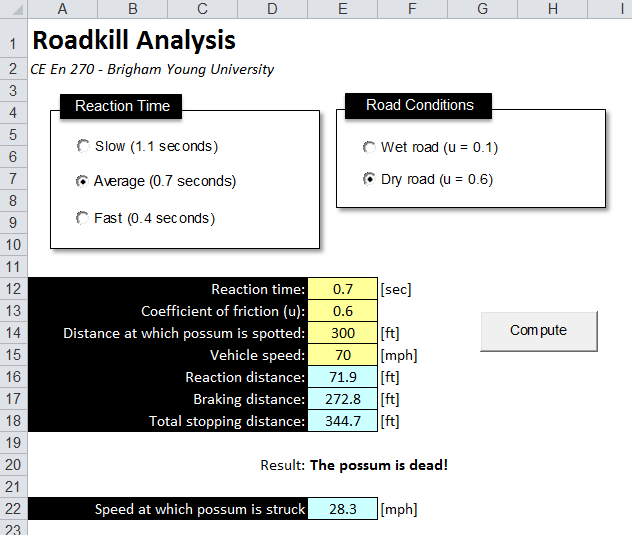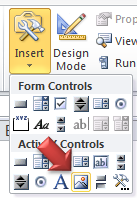In this scenario, the user is driving down a road and specifies the constant speed at which he/she is traveling. At some point the driver spots a possum crossing the road in front of the car. The possum freezes in its tracks and the user slams on the brakes. The spreadsheet should let the user know if he/she will be able to stop in time.

It takes the average driver about 0.7 seconds to apply the brakes after seeing the need to stop; this is called the driver's reaction time. Once the brakes are applied, the car decelerates. Assuming a constant deceleration a, the braking distance x is given by:

x = -v2/(2a)

The deceleration is given by:

a = -ug

Where g is the acceleration due to gravity (32.2 ft/s2) and u is the coefficient of friction between the car's tires and the road. The total stopping distance is the sum of the distance traveled during the reaction time and the braking distance. If the total stopping distance exceeds the distance at which the possum is spotted, the possum becomes roadkill. In that case, you can also compute the speed at which the possum is struck using this formula:

where vf = final velocity (velocity on impact), vi = speed prior to braking, a = deceleration as defined above, and d = actual braking distance (i.e., distance at which possum is spotted minus the reaction distance). Solve this equation for vf.

The spreadsheet should allow the user to specify the speed of the car [mph], the distance [ft] at which the opossum is spotted, the road conditions (for wet road, u = 0.1, for dry road u = 0.6), and the driver's reaction time (fast = 0.4 seconds, average = 0.7 seconds, slow = 1.1 seconds). (Make sure you are consistent with your units!).

Your spreadsheet should include two option groups as shown above. Be sure to use a unique group name for each set of option controls. When the user clicks on one of the option controls, you should update the value in the appropriate cell (reaction time or friction coefficient).

Your calculations should be done in VB code. When the user clicks on the Compute button, you should copy the values of the four input cells to a set of variables with the type "double", perform the calculations, and put the results in the four cells shown in blue plus a text description of the outcome as shown above.

For this assignment, you will need to use the VB If statement.  The syntax of the If statement is as follows:

If (condition) Then
<code that is executed if the condition is true>
Else
<code that is executed if the condition is false>
End If

For example

If stopdistance < sightdistance Then
Range("E20") = "The possum is safe."
Range("E22") = 0
Else
Range("E20") = "The possum is dead!"
<put your code here to calculate vf>
Range("E22") = vf
End If

### Possum Images

While this is NOT required, it is fun to associate some graphics with your results. For example, you can have an image of a dead possum appear in one case and have an image of a live possum appear in the other case. This is actually quite easy to do. First of all, you will need to locate and download two images to your local drive. Then, create two image controls on your spreadsheet:Drag a rectangle somewhere on the sheet for each of the two controls. Give them descriptive names (imgLive and imgDead for example). With one of the controls selected, look in the Control Properties window and you will see a Picture property. Select the control and you will see a little button the right labelled "...". Click on this button and select the appropriate file from the two that you downloaded. This will link the image to the control. Repeat for the other control.

To turn the controls on and off, you simply need to modify the Visible property of the controls. It is a Boolean field so you just toggle it between True and False. You could simply modify the If statement shown above as follows:

If stopdistance < distance1 Then
Range(E20) = "The possum is safe."
Range(E22) = 0
imgLive.Visible = True

Else
Range(E20) = "The possum is dead!"
<put your code here to calculate vf>
Range(E22) = vf